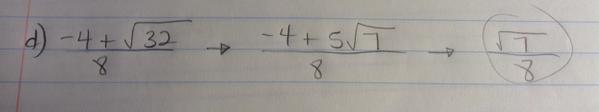CategoriesCool mistake!

A meta-question: what can we learn from a mistake like this, compared to a set of classwork on simplifying this expression?

## 3 replies on “Simplifying Radicals”

Chester Drawssays:

I am utterly flummoxed by the first step. I just can’t get 5s and 7s into 32. Unless he misread the 32 as 35 and got 3 x 5 = 32

A boy of mine recently did 3x – 2 ( = 1x ) = x, so I think the last step might be the same logic. This arises, as far as I can tell, from thinking the radical/unknown is somehow separate from the coefficient, and so the operations work on them separately. (Usually we see that with 4x – x = 4.)

Howard Phillipssays:

32=25+7, so root(32)=root(25+7)=5*root(7)

Tiffany Taosays:

I would like to ask what age this student was, and what grade that this subtraction question was asked? Actually this student makes two different mistakes. The student may know that how to do the multiplication in some way, but he or she may not understand what the root signs mean. rtsq 32 should equal to 4rtsq2 instead of 5rtsq7. The student think that 5^2+7=32, but acctually the relationship between the number in and outside the root sign is multiplication. The second mistake the student makes is that he or she do the addition first: -4+5=1. He or she should do the root calculation first and then do the addition.